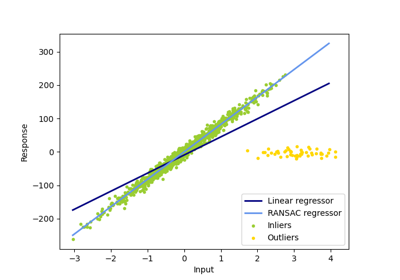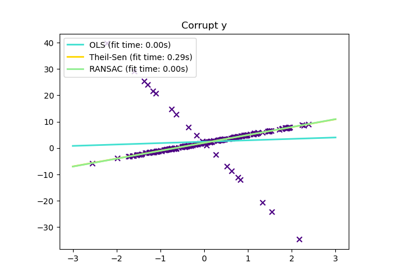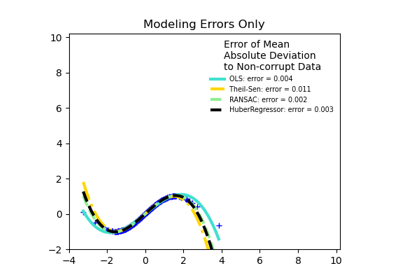# sklearn.linear_model.RANSACRegressor¶

class sklearn.linear_model.RANSACRegressor(base_estimator=None, *, min_samples=None, residual_threshold=None, is_data_valid=None, is_model_valid=None, max_trials=100, max_skips=inf, stop_n_inliers=inf, stop_score=inf, stop_probability=0.99, loss='absolute_loss', random_state=None)[source]

RANSAC (RANdom SAmple Consensus) algorithm.

RANSAC is an iterative algorithm for the robust estimation of parameters from a subset of inliers from the complete data set.

Read more in the User Guide.

Parameters
base_estimatorobject, optional

Base estimator object which implements the following methods:

• fit(X, y): Fit model to given training data and target values.

• score(X, y): Returns the mean accuracy on the given test data, which is used for the stop criterion defined by stop_score. Additionally, the score is used to decide which of two equally large consensus sets is chosen as the better one.

• predict(X): Returns predicted values using the linear model, which is used to compute residual error using loss function.

If base_estimator is None, then base_estimator=sklearn.linear_model.LinearRegression() is used for target values of dtype float.

Note that the current implementation only supports regression estimators.

min_samplesint (>= 1) or float ([0, 1]), optional

Minimum number of samples chosen randomly from original data. Treated as an absolute number of samples for min_samples >= 1, treated as a relative number ceil(min_samples * X.shape) for min_samples < 1. This is typically chosen as the minimal number of samples necessary to estimate the given base_estimator. By default a sklearn.linear_model.LinearRegression() estimator is assumed and min_samples is chosen as X.shape + 1.

residual_thresholdfloat, optional

Maximum residual for a data sample to be classified as an inlier. By default the threshold is chosen as the MAD (median absolute deviation) of the target values y.

is_data_validcallable, optional

This function is called with the randomly selected data before the model is fitted to it: is_data_valid(X, y). If its return value is False the current randomly chosen sub-sample is skipped.

is_model_validcallable, optional

This function is called with the estimated model and the randomly selected data: is_model_valid(model, X, y). If its return value is False the current randomly chosen sub-sample is skipped. Rejecting samples with this function is computationally costlier than with is_data_valid. is_model_valid should therefore only be used if the estimated model is needed for making the rejection decision.

max_trialsint, optional

Maximum number of iterations for random sample selection.

max_skipsint, optional

Maximum number of iterations that can be skipped due to finding zero inliers or invalid data defined by is_data_valid or invalid models defined by is_model_valid.

New in version 0.19.

stop_n_inliersint, optional

Stop iteration if at least this number of inliers are found.

stop_scorefloat, optional

Stop iteration if score is greater equal than this threshold.

stop_probabilityfloat in range [0, 1], optional

RANSAC iteration stops if at least one outlier-free set of the training data is sampled in RANSAC. This requires to generate at least N samples (iterations):

N >= log(1 - probability) / log(1 - e**m)


where the probability (confidence) is typically set to high value such as 0.99 (the default) and e is the current fraction of inliers w.r.t. the total number of samples.

lossstring, callable, optional, default “absolute_loss”

String inputs, “absolute_loss” and “squared_loss” are supported which find the absolute loss and squared loss per sample respectively.

If loss is a callable, then it should be a function that takes two arrays as inputs, the true and predicted value and returns a 1-D array with the i-th value of the array corresponding to the loss on X[i].

If the loss on a sample is greater than the residual_threshold, then this sample is classified as an outlier.

New in version 0.18.

random_stateint, RandomState instance, default=None

The generator used to initialize the centers. Pass an int for reproducible output across multiple function calls. See Glossary.

Attributes
estimator_object

Best fitted model (copy of the base_estimator object).

n_trials_int

Number of random selection trials until one of the stop criteria is met. It is always <= max_trials.

Boolean mask of inliers classified as True.

n_skips_no_inliers_int

Number of iterations skipped due to finding zero inliers.

New in version 0.19.

n_skips_invalid_data_int

Number of iterations skipped due to invalid data defined by is_data_valid.

New in version 0.19.

n_skips_invalid_model_int

Number of iterations skipped due to an invalid model defined by is_model_valid.

New in version 0.19.

References

1

https://en.wikipedia.org/wiki/RANSAC

2

https://www.sri.com/sites/default/files/publications/ransac-publication.pdf

3

http://www.bmva.org/bmvc/2009/Papers/Paper355/Paper355.pdf

Examples

>>> from sklearn.linear_model import RANSACRegressor
>>> from sklearn.datasets import make_regression
>>> X, y = make_regression(
...     n_samples=200, n_features=2, noise=4.0, random_state=0)
>>> reg = RANSACRegressor(random_state=0).fit(X, y)
>>> reg.score(X, y)
0.9885...
>>> reg.predict(X[:1,])
array([-31.9417...])


Methods

 fit(X, y[, sample_weight]) Fit estimator using RANSAC algorithm. get_params([deep]) Get parameters for this estimator. Predict using the estimated model. score(X, y) Returns the score of the prediction. set_params(**params) Set the parameters of this estimator.
__init__(base_estimator=None, *, min_samples=None, residual_threshold=None, is_data_valid=None, is_model_valid=None, max_trials=100, max_skips=inf, stop_n_inliers=inf, stop_score=inf, stop_probability=0.99, loss='absolute_loss', random_state=None)[source]

Initialize self. See help(type(self)) for accurate signature.

fit(X, y, sample_weight=None)[source]

Fit estimator using RANSAC algorithm.

Parameters
Xarray-like or sparse matrix, shape [n_samples, n_features]

Training data.

yarray-like of shape (n_samples,) or (n_samples, n_targets)

Target values.

sample_weightarray-like of shape (n_samples,), default=None

Individual weights for each sample raises error if sample_weight is passed and base_estimator fit method does not support it.

New in version 0.18.

Raises
ValueError

If no valid consensus set could be found. This occurs if is_data_valid and is_model_valid return False for all max_trials randomly chosen sub-samples.

get_params(deep=True)[source]

Get parameters for this estimator.

Parameters
deepbool, default=True

If True, will return the parameters for this estimator and contained subobjects that are estimators.

Returns
paramsmapping of string to any

Parameter names mapped to their values.

predict(X)[source]

Predict using the estimated model.

This is a wrapper for estimator_.predict(X).

Parameters
Xnumpy array of shape [n_samples, n_features]
Returns
yarray, shape = [n_samples] or [n_samples, n_targets]

Returns predicted values.

score(X, y)[source]

Returns the score of the prediction.

This is a wrapper for estimator_.score(X, y).

Parameters
Xnumpy array or sparse matrix of shape [n_samples, n_features]

Training data.

yarray, shape = [n_samples] or [n_samples, n_targets]

Target values.

Returns
zfloat

Score of the prediction.

set_params(**params)[source]

Set the parameters of this estimator.

The method works on simple estimators as well as on nested objects (such as pipelines). The latter have parameters of the form <component>__<parameter> so that it’s possible to update each component of a nested object.

Parameters
**paramsdict

Estimator parameters.

Returns
selfobject

Estimator instance.

## Examples using sklearn.linear_model.RANSACRegressor¶